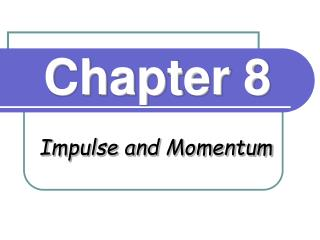# Chapter 8 - PowerPoint PPT PresentationDownload PresentationChapter 8

Chapter 8
Download Presentation## Chapter 8

- - - - - - - - - - - - - - - - - - - - - - - - - - - E N D - - - - - - - - - - - - - - - - - - - - - - - - - - -
##### Presentation Transcript

1. Chapter 8 Impulse and Momentum

2. Momentum and Collisions • This chapter is concerned with inertia and motion. Momentum helps us understand collisions. • Elastic Collisions - objects rebound • Inelastic Collisions - object stick together an usually become distorted and generate heat

3. Momentum • Momentum = mass ´ velocity • p = mv • Momentum is a vector quantity.

4. Large Momentum Examples • Huge ship moving at a small velocity • High velocity bullet P = Mv P = mv

5. Momentum Examples • A large truck has more momentum than a car moving at the same speed because it has a greater mass. • Which is more difficult to slow down? The car or the large truck?

6. Impulse • Newton’s Second Law can read SF = ma = m(Dv/Dt) = (Dmv)/(Dt) = (Dp/ Dt) Rearranging, Impulse = Dp = FDt

7. When Force is Limited • Apply a force for a long time. • Examples: • Follow through on a golf swing. • Pushing a car. FDt

8. Make it Bounce Dp = p2 - p1 = -p1 - p1 = -2p1 p1 p2 = -p1

9. Minimize the Force • Increase Dt • Catching a ball • Bungee jumping FDt

10. Maximize Momentum Change Apply a force for a short time. • Examples: • Boxing • Karate FDt

11. Conservation of Momentum • This means that the momentum doesn’t change. • Recall that SF t = D(mv), so SF = 0 • In this equation, F is the "external force." • Internal forces cannot cause a change in momentum.

12. Examples • Example 1: a bullet fired from a rifle • Example 2: a rocket in space

13. m1 m2 m1 m2 Collisions Before After

14. M M M M Inelastic Collisions v = 10 v = 0 Before Collision p = Mv v’ = 5 After Collision p = 2Mv’ Mv = 2Mv’ v’ = ½ v

15. Elastic Collisions Conserve Energy and Momentum Before Collision Equal masses Case 1: Case 2: M > M Case 3: M < M

16. Coefficient of Restitution • For perfectly elastic collisions e = 1. • If the two object stick together, e = 0. • Otherwise 0 < e < 1.

17. Center of Mass

18. On to problems...